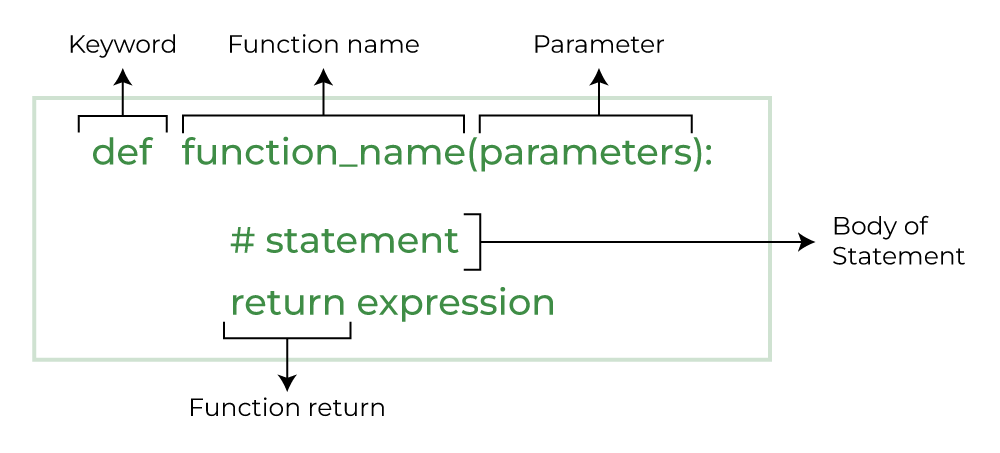# Learning Python with Advent of Code Walkthroughs

Dazbo's Advent of Code solutions, written in Python

# The Python Journey - Functions## Functions

### Overview

A function is a way of taking some code and making it callable. This allows us to implement chunks of code that we want to re-use. It also helps us break down our code into manageable and readable units of functionality.

The general syntax is:

``````def my_function(some_arg, some_other_arg):
""" Some docstring """
some_result = do_some_stuff(some_arg)
ret_value = do_some_other_stuff(some_other_arg, some_result)
return ret_value
``````
• The `def` keyword binds a function body (i.e. the code) to a name, such that a function is simply an object. Thus, a function is a callable object.
• After a function has been defined, we can call our function from another part of our code.
• Function arguments are passed in the brackets. Functions do not always require arguments.
• It is useful to include a docstring, i.e. brief documentation that explains what the function does, and how to use it.
• A function does not always need to return a value. But often, a function does something, and then returns a value as output. If no return value is explicitly specified, the function will always return `None`.
• Functions can return multiple values, as a `tuple`. Whenever more than one value is returned by a function, it is automatically converted to a tuple. For example:
``````def min_and_max(items):
""" Takes a list of values, and returns the smallest and largest values from the list.
Returns: a tuple, composed of the min and max values from the list """
return min(items), max(items)

print(min_and_max([50,5,20,30]))
``````

Output:

``````(5, 50)
``````

Note how the output is actually just a single tuple, containing our two values.

### Fibonacci Example

The Fibonacci sequence is an infinite sequence where each value is the sum of the previous two values.

``````1, 1, 2, 3, 5, 8, 13, 21, etc
``````

Here I implement a function that generates the sequence with n iterations.

``````def fib(n):
""" Generate the Fibonacci Sequence for n iterations """
ret_val = []
if (n==0):
return ret_val

a = 0
b = 1

for iteration in range(n):
ret_val.append(f"Iteration {iteration}: value={str(b)}")
a, b = b, a+b

return ret_val

# Test our code
try:
iterations = int(input("How many Fibonacci numbers would you like? "))
values = fib(iterations)
for v in values:
print(v)
except ValueError:
print("You didn't enter a number, you muppet.")
``````

Some points to note:

In place swapping is cool!

``````temp = a
a = b
b = a + temp
``````

We can write this:

``````a, b = b, a+b
``````
• To handle bad input, I’m using exceptions. Check out my page on this, here.
• I’m using `range(n)` in combination with a `for` loop so that we can iterate over the loop `n` times.

### Lambda Functions

A lambda function is a small anonymous function that contains only a single expression. When we say “anonymous”, we mean that the function is not explicitly defined using `def`. Instead, it is simply defined as an expression, at the point it is required.

A lambda function can have zero, one or many arguments. But it can only perform a single statement, and this statement must be an expression that evaluates to a result.

Clear as mud?

This will help:

``````# Here we define a function explicitly, using the def keyword
return x + y

# But this lambda function is equivalent.
# The accepted arguments are always placed before the colon.
# The expression that returns is written after the colon.
lambda x, y: x + y
``````

Let’s try using a lambda function to do something useful.

Imagine we have a list of names, where each name is given in the format “firstname lastname”. We want to sort the list based on the lastname.

We can do this with a lambda:

``````people = [
"Bob Pleb",
"Duncan Idaho",
"Fred Planck",
"Oliver Smith",
"Russ Martin",

# sort using lambda that splits each name and returns the last name
sorted_people = sorted(people, key=lambda name: name.split()[-1])
print("\n".join(sorted_people))
``````

Our lambda function is simply this:

``````lambda name: name.split()[-1]
``````

It expects a single parameter, called name. It splits this name, and then returns the last word from the split. Hence, if `name` is `"firstname lastname"`, then the result of `name.split()[-1]` will be `"lastname"`.

We pass this lambda function into our `sorted()` function, which then applies the lambda function to every member of this list.

Thus, the output is sorted in alphabetically last name order, as required:

``````Zoltan Adler
Duncan Idaho
Russ Martin
Fred Planck
Bob Pleb
Oliver Smith
``````

Consequently, lambda functions are extremely useful as parameters to any functions that work on collections, such as `min`, `max`, `sorted`, `map`, `filter`, and `reduce`.

See: